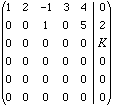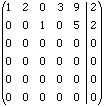Hi,

My name is Will. Level: (10-12)

I have been having some problem with the following question for some time. I would appreciate any help on solving the problem or a solution.

Q: Assume that a system of equations in the unknowns x1, x2, x3, x4 and x5 when converted to row echelon form givesSolve, if possible for x1, x2, x3, x4 and x5 in the two cases

(a) k = -3
(b) k = 0.

Hi Will,

If k = -3 then the third row of your matrix represents the equation

0 x1 + 0 x2 + 0 x3 + 0 x4 + 0 x5 = -3

This is clearly impossible and thus if k = -3 then the system of equations has no solution.

If k = 0 however the system has infinitely many solutions. To describe these solutions I would first put the matrix in reduced row echelon form by adding the second row to the first row to getThe two remaining, non-zero rows represent the equations

 x1 = 2 -2 x2 -3 x4 -9 x5 x3 = 2 -5 x5

This gives infinitely many solutions since you are free to assign arbitrary values to x3, x4 and x5 and then compute values for x1 and x3 to form a solution.

Cheers,
Penny
Go to Math Central